# NERD force field

 Molecule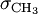$\sigma_{\mathrm {CH}_3}$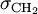$\sigma_{\mathrm {CH}_2}$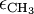$\epsilon_{\mathrm {CH}_3}$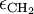$\epsilon_{\mathrm {CH}_2}$ ethane 3.825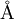$\mathrm{\AA}$ 3.93$\mathrm{\AA}$ 100.6 K propane 3.857$\mathrm{\AA}$ 3.93$\mathrm{\AA}$ 102.6 K 45.8 K butane and longer 3.91$\mathrm{\AA}$ 3.93$\mathrm{\AA}$ 104.0 K 45.8 K